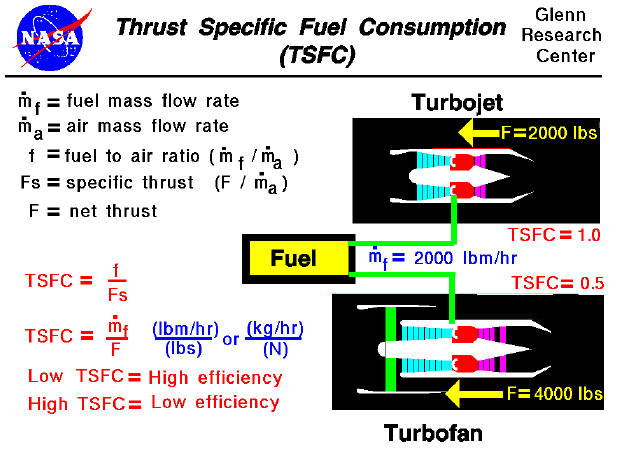The interactive Java applet EngineSim is now available. You can study the effects of any engine component's performance on fuel consumption and compare the efficiency of various types of turbine engines.

To move an airplane through the air, a propulsion system is used to generate thrust.

The amount of thrust an engine generates is important. But the amount of fuel used to generate that thrust is sometimes more important, because the airplane has to lift and carry the fuel throughout the flight. Engineers use an efficiency factor, called thrust specific fuel consumption, to characterize an engine's fuel efficiency. "Thrust specific fuel consumption" is quite a mouthful, so engineers usually just call it the engine's TSFC. What does TSFC mean?

The fuel consumption of TSFC is "how much fuel the engine burns each hour." The specific of TSFC is a scientific term meaning "divided by mass or weight." In this case, specific means "per pound (Newton) of thrust." The thrust of TSFC is included to indicate that we are talking about gas turbine engines. There is a corresponding brake specific fuel consumption (BSFC) for engines that produce shaft power. Gathering all the terms together, TSFC is the mass of fuel burned by an engine in one hour divided by the thrust that the engine produces. The units of this efficiency factor are mass per time divided by force (in English units, pounds mass per hour per pound; in metric units, kilograms per hour per Newton).

Mathematically, TSFC equals the engine fuel mass flow rate (mdot f) divided by the thrust (F)

TSFC = mdot f / F

If we divide both numerator and denominator by the engine airflow (mdot 0), obtain another form of the equation in terms of the fuel to air ratio (f), and the specific thrust (Fs).

TSFC = f / Fs

Engineers use the TSFC factor several different ways. If we compare the TSFC for two engines, the engine with the lower TSFC is the more fuel efficient engine. Let's consider two examples:

• Suppose we had two Engines, A and B, that produced the same amount of thrust. And suppose that Engine A uses only half the fuel per hour that Engine B uses. We would then say that Engine A is more fuel efficient than Engine B. If we compute the TSFC for Engines A and B, the TSFC of Engine A is one half the value of Engine B.
• Looking at it another way, suppose we had two Engines, C and D, and we fed the same amount of fuel per hour to each of them. Suppose Engine C produces twice the thrust of Engine D. Then we are getting more thrust from Engine C for the same amount of fuel, and we would say that Engine C is more fuel efficient. Again, if we compute the TSFC for Engines C and D, the TSFC of Engine C is one half the value of Engine D.

Let's look at the second example with some numerical values. In this case we are comparing a turbojet engine and a turbofan engine. The engines are being fed from a fuel tank that delivers 2000 pounds mass per hour to each engine. The turbojet produces 2000 pounds of thrust, while the turbofan produces 4000 pounds of thrust. Computing the TSFC for each engine shows that the TSFC of the turbojet is equal to 1.0 (pounds mass/hour/pound) while the TSFC of the turbofan is 0.5 (pounds mass/ hour/pound). The turbofan, with a lower TSFC, is more fuel efficient. The values of 1.0 for a turbojet and 0.5 for a turbofan are typical sea level static values. The value of TSFC for a given engine will vary with speed and altitude, because the efficiency of the engine changes with atmospheric conditions.

TSFC provides important information about the performance of a given engine. A turbojet with an afterburner produces more thrust than a plain turbojet. If the TSFC were the same (1.0) for the two engines, in order to increase thrust, we would have to increase the fuel flow rate by an equivalent amount. For example,

Initial thrust = 2000 pounds
Thrust with afterburner = 3000 pounds
TSFC = 1.0
Fuel flow rate = 3000 pounds per hour.

But a turbojet with an afterburner has a typical TSFC value of 1.5. This says that adding the afterburner, although it produces more thrust, costs much more fuel for each pound of thrust added. For example,

Initial thrust = 2000 pounds
Thrust with afterburner = 3000 pounds
TSFC = 1.5
Fuel flow rate = 4500 pounds per hour.

Engineers use the TSFC for a given engine to figure out how much fuel is required for an aircraft to perform a given mission. If the TSFC = 0.5, and we need 5000 pounds of thrust for two hours, we can easily compute the amount of fuel required. For example,

5000 pounds x 0.5 pound mass/hour/pound x 2 hours = 5000 pound mass of fuel.

Guided Tours